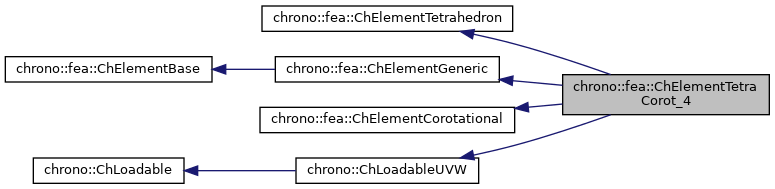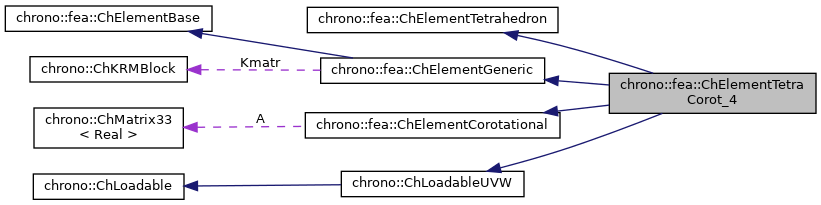chrono::fea::ChElementTetraCorot_4 Class Reference

## Description

Tetrahedron FEA element with 4 nodes.

This is a classical element with linear displacement, hence with constant stress and constant strain. It can be easily used for 3D FEA problems.

#include <ChElementTetraCorot_4.h>

Inheritance diagram for chrono::fea::ChElementTetraCorot_4:[legend]
Collaboration diagram for chrono::fea::ChElementTetraCorot_4:[legend]

## Public Types

using ShapeVector = ChMatrixNM< double, 1, 4 >

## Public Member Functions

virtual int GetNnodes () override
Gets the number of nodes used by this element.

virtual int GetNdofs () override
Gets the number of coordinates in the field used by the referenced nodes. More...

virtual int GetNodeNdofs (int n) override
Get the number of coordinates from the n-th node that are used by this element. More...

double GetVolume ()

virtual std::shared_ptr< ChNodeFEAbaseGetNodeN (int n) override
Access the nth node.

virtual std::shared_ptr< ChNodeFEAxyzGetTetrahedronNode (int n) override
Return the specified tetrahedron node (0 <= n <= 3).

virtual void SetNodes (std::shared_ptr< ChNodeFEAxyz > nodeA, std::shared_ptr< ChNodeFEAxyz > nodeB, std::shared_ptr< ChNodeFEAxyz > nodeC, std::shared_ptr< ChNodeFEAxyz > nodeD)

virtual void Update () override
Update element at each time step.

void ShapeFunctions (ShapeVector &N, double r, double s, double t)
Fills the N shape function matrix with the values of shape functions at r,s,t 'volume' coordinates, where r=1 at 2nd vertex, s=1 at 3rd, t = 1 at 4th. More...

virtual void GetStateBlock (ChVectorDynamic<> &mD) override
Fills the D vector (displacement) with the currentfield values at the nodes of the element, with proper ordering. More...

double ComputeVolume ()

virtual void ComputeStiffnessMatrix ()
Computes the local STIFFNESS MATRIX of the element: K = Volume * [B]' * [D] * [B].

virtual void UpdateRotation () override
compute large rotation of element for corotational approach

virtual void ComputeKRMmatricesGlobal (ChMatrixRef H, double Kfactor, double Rfactor=0, double Mfactor=0) override
Sets H as the global stiffness matrix K, scaled by Kfactor. More...

virtual void ComputeInternalForces (ChVectorDynamic<> &Fi) override
Computes the internal forces (ex. More...

void SetMaterial (std::shared_ptr< ChContinuumElastic > my_material)
Set the material of the element.

std::shared_ptr< ChContinuumElasticGetMaterial ()

const ChMatrixDynamicGetMatrB () const
Get the partial derivatives matrix MatrB and the StiffnessMatrix.

const ChMatrixDynamicGetStiffnessMatrix () const

ChStrainTensor GetStrain ()
Returns the strain tensor (note that the tetrahedron 4 nodes is a linear element, thus the strain is constant in the entire volume). More...

ChStressTensor GetStress ()
Returns the stress tensor (note that the tetrahedron 4 nodes is a linear element, thus the stress is constant in the entire volume). More...

void ComputeNodalMass () override
This function computes and adds corresponding masses to ElementBase member m_TotalMass.

Gets the number of DOFs affected by this element (position part)

Gets the number of DOFs affected by this element (speed part)

virtual void LoadableGetStateBlock_x (int block_offset, ChState &mD) override
Gets all the DOFs packed in a single vector (position part)

virtual void LoadableGetStateBlock_w (int block_offset, ChStateDelta &mD) override
Gets all the DOFs packed in a single vector (speed part)

virtual void LoadableStateIncrement (const unsigned int off_x, ChState &x_new, const ChState &x, const unsigned int off_v, const ChStateDelta &Dv) override
Increment all DOFs using a delta.

virtual int Get_field_ncoords () override
Number of coordinates in the interpolated field: here the {x,y,z} displacement.

virtual int GetSubBlocks () override
Get the number of DOFs sub-blocks.

virtual unsigned int GetSubBlockOffset (int nblock) override
Get the offset of the specified sub-block of DOFs in global vector.

virtual unsigned int GetSubBlockSize (int nblock) override
Get the size of the specified sub-block of DOFs in global vector.

virtual bool IsSubBlockActive (int nblock) const override
Check if the specified sub-block of DOFs is active.

virtual void LoadableGetVariables (std::vector< ChVariables * > &mvars) override
Get the pointers to the contained ChVariables, appending to the mvars vector.

virtual void ComputeNF (const double U, const double V, const double W, ChVectorDynamic<> &Qi, double &detJ, const ChVectorDynamic<> &F, ChVectorDynamic<> *state_x, ChVectorDynamic<> *state_w) override
Evaluate N'*F , where N is some type of shape function evaluated at U,V,W coordinates of the volume, each ranging in 0..+1 F is a load, N'*F is the resulting generalized load Returns also det[J] with J=[dx/du,..], that might be useful in gauss quadrature. More...

virtual double GetDensity () override
This is needed so that it can be accessed by ChLoaderVolumeGravity.

virtual bool IsTetrahedronIntegrationNeeded () override
If true, use quadrature over u,v,w in [0..1] range as tetrahedron volumetric coords, with z=1-u-v-w otherwise use quadrature over u,v,w in [-1..+1] as box isoparametric coords.Public Member Functions inherited from chrono::fea::ChElementGeneric
ChKblockGenericKstiffness ()
Access the proxy to stiffness, for sparse solver.

virtual void EleIntLoadResidual_F (ChVectorDynamic<> &R, const double c) override
(This is a default (a bit unoptimal) book keeping so that in children classes you can avoid implementing this EleIntLoadResidual_F function, unless you need faster code)

virtual void EleIntLoadResidual_Mv (ChVectorDynamic<> &R, const ChVectorDynamic<> &w, const double c) override
(This is a default (VERY UNOPTIMAL) book keeping so that in children classes you can avoid implementing this EleIntLoadResidual_Mv function, unless you need faster code.)

virtual void EleIntLoadResidual_F_gravity (ChVectorDynamic<> &R, const ChVector<> &G_acc, const double c) override
(This is a default (VERY UNOPTIMAL) book keeping so that in children classes you can avoid implementing this EleIntLoadResidual_F_gravity function, unless you need faster code. More...

virtual void ComputeGravityForces (ChVectorDynamic<> &Fg, const ChVector<> &G_acc) override
(This is the default implementation (POTENTIALLY INEFFICIENT) so that in children classes you can avoid implementing this ComputeGravityForces() function, unless you need faster code.) This fallback implementation uses a temp ChLoaderGravity that applies the load to elements only if they are inherited by ChLoadableUVW so it can use GetDensity() and Gauss quadrature.

virtual void ComputeMmatrixGlobal (ChMatrixRef M) override
Returns the global mass matrix. More...

virtual void InjectKRMmatrices (ChSystemDescriptor &mdescriptor) override
Tell to a system descriptor that there are item(s) of type ChKblock in this object (for further passing it to a solver)

virtual void KRMmatricesLoad (double Kfactor, double Rfactor, double Mfactor) override
Adds the current stiffness K and damping R and mass M matrices in encapsulated ChKblock item(s), if any. More...

virtual void VariablesFbLoadInternalForces (double factor=1.) override
Adds the internal forces, expressed as nodal forces, into the encapsulated ChVariables, in the 'fb' part: qf+=forces*factor (This is a default (a bit unoptimal) book keeping so that in children classes you can avoid implementing this VariablesFbLoadInternalForces function, unless you need faster code)

virtual void VariablesFbIncrementMq () override
Adds M*q (internal masses multiplied current 'qb') to Fb, ex. More...Public Member Functions inherited from chrono::fea::ChElementBase
virtual void EleDoIntegration ()
This is optionally implemented if there is some internal state that requires integration.Public Member Functions inherited from chrono::fea::ChElementCorotational
ChMatrix33Rotation ()
Access the cumulative rotation matrix of the element. More...Public Member Functions inherited from chrono::ChLoadableUVW
virtual bool IsTrianglePrismIntegrationNeeded ()
If true, use quadrature over u,v in [0..1] range as triangle natural coords (with z=1-u-v), and use linear quadrature over w in [-1..+1], otherwise use default quadrature over u,v,w in [-1..+1] as box isoparametric coords.Protected Attributes inherited from chrono::fea::ChElementGeneric
ChKblockGeneric KmatrProtected Attributes inherited from chrono::fea::ChElementCorotational
ChMatrix33 A

## ◆ ComputeInternalForces()

 void chrono::fea::ChElementTetraCorot_4::ComputeInternalForces ( ChVectorDynamic<> & Fi )
overridevirtual

Computes the internal forces (ex.

the actual position of nodes is not in relaxed reference position) and set values in the Fi vector.

Implements chrono::fea::ChElementBase.

## ◆ ComputeKRMmatricesGlobal()

 void chrono::fea::ChElementTetraCorot_4::ComputeKRMmatricesGlobal ( ChMatrixRef H, double Kfactor, double Rfactor = 0, double Mfactor = 0 )
overridevirtual

Sets H as the global stiffness matrix K, scaled by Kfactor.

Optionally, also superimposes global damping matrix R, scaled by Rfactor, and global mass matrix M multiplied by Mfactor.

Implements chrono::fea::ChElementBase.

## ◆ ComputeNF()

 void chrono::fea::ChElementTetraCorot_4::ComputeNF ( const double U, const double V, const double W, ChVectorDynamic<> & Qi, double & detJ, const ChVectorDynamic<> & F, ChVectorDynamic<> * state_x, ChVectorDynamic<> * state_w )
overridevirtual

Evaluate N'*F , where N is some type of shape function evaluated at U,V,W coordinates of the volume, each ranging in 0..+1 F is a load, N'*F is the resulting generalized load Returns also det[J] with J=[dx/du,..], that might be useful in gauss quadrature.

Parameters
 U parametric coordinate in volume V parametric coordinate in volume W parametric coordinate in volume Qi Return result of N'*F here, maybe with offset block_offset detJ Return det[J] here F Input F vector, size is = n.field coords. state_x if != 0, update state (pos. part) to this, then evaluate Q state_w if != 0, update state (speed part) to this, then evaluate Q

## ◆ GetNdofs()

 virtual int chrono::fea::ChElementTetraCorot_4::GetNdofs ( )
inlineoverridevirtual

Gets the number of coordinates in the field used by the referenced nodes.

This is for example the size (n.of rows/columns) of the local stiffness matrix.

Implements chrono::fea::ChElementBase.

## ◆ GetNodeNdofs()

 virtual int chrono::fea::ChElementTetraCorot_4::GetNodeNdofs ( int n )
inlineoverridevirtual

Get the number of coordinates from the n-th node that are used by this element.

Note that this may be different from the value returned by GetNodeN(n)->Get_ndof_w();

Implements chrono::fea::ChElementBase.

## ◆ GetStateBlock()

 void chrono::fea::ChElementTetraCorot_4::GetStateBlock ( ChVectorDynamic<> & mD )
overridevirtual

Fills the D vector (displacement) with the currentfield values at the nodes of the element, with proper ordering.

If the D vector has not the size of this->GetNdofs(), it will be resized.For corotational elements, field is assumed in local reference!

Implements chrono::fea::ChElementBase.

## ◆ GetStrain()

 ChStrainTensor chrono::fea::ChElementTetraCorot_4::GetStrain ( )

Returns the strain tensor (note that the tetrahedron 4 nodes is a linear element, thus the strain is constant in the entire volume).

The tensor is in the original undeformed unrotated reference.

## ◆ GetStress()

 ChStressTensor chrono::fea::ChElementTetraCorot_4::GetStress ( )

Returns the stress tensor (note that the tetrahedron 4 nodes is a linear element, thus the stress is constant in the entire volume).

The tensor is in the original undeformed unrotated reference.

## ◆ ShapeFunctions()

 void chrono::fea::ChElementTetraCorot_4::ShapeFunctions ( ShapeVector & N, double r, double s, double t )

Fills the N shape function matrix with the values of shape functions at r,s,t 'volume' coordinates, where r=1 at 2nd vertex, s=1 at 3rd, t = 1 at 4th.

All ranging in [0...1]. The last (u, u=1 at 1st vertex) is computed form the first 3 as 1.0-r-s-t. NOTE! actually N should be a 3row, 12 column sparse matrix, as N = [n1*eye(3) n2*eye(3) n3*eye(3) n4*eye(3)]; , but to avoid wasting zero and repeated elements, here it stores only the n1 n2 n3 n4 values in a 1 row, 4 columns matrix.

The documentation for this class was generated from the following files:
• /builds/uwsbel/chrono/src/chrono/fea/ChElementTetraCorot_4.h
• /builds/uwsbel/chrono/src/chrono/fea/ChElementTetraCorot_4.cpp